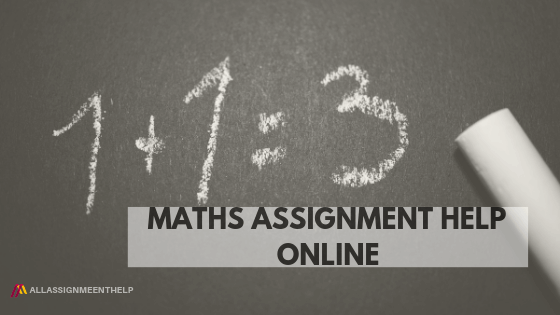Assignment Help Online

# Maths assignment help onlineIn the previous article we explored multiple area of maths assignment help online. There is always something about math assignment  that makes students feel fidgety. There are many students who are good at math,but  there will always be some assignments involving concepts that would require an expert’s help. This is where AAH- your academic hand comes into picture to provide you maths assignment help online.

Algebra assignment help online:

Lets us discuss what is algebra about and how important is the algebra assignment help is. Sometimes simple equality problems become headache. If i take an example and try to solve it using the basic rules of inequality we will see how easy and interesting math assignment help online can be. Lets take an example and start with the very basic concept of inequality.

Find the value of x that satisfies the following inequality : (x+1) > 0

Most of the students will be able to find the value of x for which the equation holds true. i.e if we substitute any value of x > -1 left hand side of the equation will always be greater than zero. Now, lets make this inequality a bit more interesting.

Find the value of x that satisfies the following inequality : ( x+1)/(x -1 )^2 > 0

Here we have an additional value in the denominator, which is (x-1)^2 . If we use our mathematical aptitude, we will see that this equation is same as the last one. Let us see how it is same as the last one.

We know a perfect square cannot be negative and if we multiply two sides of an inequality with a positive number the sign of inequality doesn’t change. For example , 4 > 3 and if we multiple both sides by 2 , new inequality will be 8 >6 ,so the inequality holds. On the other hand if we multiply both sides by -1 the sign will get reversed. For example, -4 < -3 ( after multiplying it by -1 ).

Let us take our equation again. As I said the sign of the inequality will hold on multiplication with a positive quantity, hence on multiplication with (x-1)^2 we can safely say that (x+1) > 0 * (x-1)^2 or (x+1) >0.

So students must be convinced here. However, we have missed one of the basic concept of maths assignment help online.  If we go by the last solution we can say that for x> -1 this equation holds. Let me disapprove this solution. What if we take x = 1 ?

This will lead to (x+1)/0 , and any number divided by zero becomes not defined, hence we have to eliminate those points for which the denominator becomes zero. Therefore the solution to this equation would be x > -1 and x != 1.

If we keep these basic concepts of maths assignment help online in mind we can easily deal with mathematics homework. If you have problems with the basic fundamental of maths assignment help online our writers can help you. We provide detailed solution to your problems without missing out any important information. Apart from maths assignment help online, our writers actively provide help with the assignments of the following subjects:

Apart from the offline online assignment help, we have started providing online assignment services as well. We understand the  money constraint students have therefore we are very much considerate and provide affordable assignment services online. Apart from the affordable assignment service we also have started the free assignment service online. You can post your query on our forum where our tutors are active. The turnaround time is minimal. Thank you for your time !